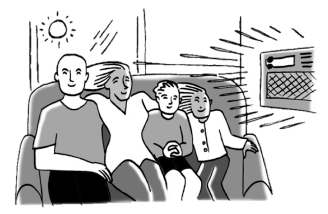### Home > CCA2 > Chapter 4 > Lesson 4.2.1 > Problem4-74

4-74.Your family plans to buy a new air conditioner. They can buy the Super Cool X1400 for $\800$, or they can buy the Efficient Energy X2000 for $\1200$. Both models will cool your home equally well, but the Efficient Energy model is less expensive to operate. The Super Cool X1400 will cost $\60$ per month to operate, while the Efficient Energy X2000 costs only $\40$ per month to operate.

1. Write an equation to represent the cost of buying and operating the Super Cool X1400 where $C=$ cost and $m=$ months.

$\text{C}=800+60m$

2. Write an equation to represent the cost of buying and operating the Efficient Energy X2000.

See part (a).

3. How many months would your family have to use the Efficient Energy model to compensate for the additional cost of the original purchase?

Set the two cost expressions equal to each other and solve algebraically.

4. Figuring your family will only use the air conditioner for $4$ months each year, how many years will you have to wait to start saving money overall?

Look at your answer to part (c). According to this problem, how many months of active use translate into one year?
Remember, the air conditioner will not be on for the whole year.